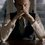# Need help in proof of 2 very important theorems!

Well, if anyone starts learning electricity and magnetism(or in particular Vol.2 of Feynman lectures, as that is my site of learning), one always gets these 2 important theorems for sure -

$\displaystyle \text{1. Gauss' Theorem -} \int_{S}{C.n dS} = \int_{V}{\nabla.C dV}$ such that C is any vector, S area, V volume and $$\nabla.C$$ is the divergence of C.

$\displaystyle \text{2. Stokes' Theorem -} \int_{line}{C ds} = \int_{S}{{(\nabla \times C)}_{n} dS}$ such that C is any vector, $\int_{line}{}$ means line integral, $\nabla \times C$ is curl of C.

Now, I want proofs of both these strong theorems. Please if anyone can help me in any way, care to do so. Thanks in anticipation!Note by Kartik Sharma
6 years, 4 months ago

This discussion board is a place to discuss our Daily Challenges and the math and science related to those challenges. Explanations are more than just a solution — they should explain the steps and thinking strategies that you used to obtain the solution. Comments should further the discussion of math and science.

When posting on Brilliant:

• Use the emojis to react to an explanation, whether you're congratulating a job well done , or just really confused .
• Ask specific questions about the challenge or the steps in somebody's explanation. Well-posed questions can add a lot to the discussion, but posting "I don't understand!" doesn't help anyone.
• Try to contribute something new to the discussion, whether it is an extension, generalization or other idea related to the challenge.

MarkdownAppears as
*italics* or _italics_ italics
**bold** or __bold__ bold
- bulleted- list
• bulleted
• list
1. numbered2. list
1. numbered
2. list
Note: you must add a full line of space before and after lists for them to show up correctly
paragraph 1paragraph 2

paragraph 1

paragraph 2

[example link](https://brilliant.org)example link
> This is a quote
This is a quote
    # I indented these lines
# 4 spaces, and now they show
# up as a code block.

print "hello world"
# I indented these lines
# 4 spaces, and now they show
# up as a code block.

print "hello world"
MathAppears as
Remember to wrap math in $$ ... $$ or $ ... $ to ensure proper formatting.
2 \times 3 $2 \times 3$
2^{34} $2^{34}$
a_{i-1} $a_{i-1}$
\frac{2}{3} $\frac{2}{3}$
\sqrt{2} $\sqrt{2}$
\sum_{i=1}^3 $\sum_{i=1}^3$
\sin \theta $\sin \theta$
\boxed{123} $\boxed{123}$

Sort by:

Do you still need help with it or have you found out a way to solve it yourself ?

- 6 years, 3 months ago

Still need! That's why I have shared the set! Check out others also! Well, I have one proof with some assumptions but I want a 'proper mathematical proof'!

- 6 years, 3 months ago

Good problems should be challenging but not tedious. Unrelated to this thread but just a reminder/word of advice.

- 6 years, 3 months ago

Oh yeah! That's right. I was thinking of that while sharing them but then I thought there isn't any 'tedious' type of thing in mathematics. If calculations is what you are saying, then you have a calculator, I wouldn't mind.

- 6 years, 3 months ago

The given theorems are particular cases of a very strong theorem in differential geometry/Calculus on Manifolds, called generalized Stokes' theorem; although applied to electricity concepts. You can see any vector calculus book for particular cases proof and Michael Spicak's Calculus on Manifolds or Rudin's Principles of Mathematical Analysis for the proof of general case. As for proof corresponding to Physical case, you could refer Piyush A Kundu's Electricity textbook.

- 4 years, 5 months ago